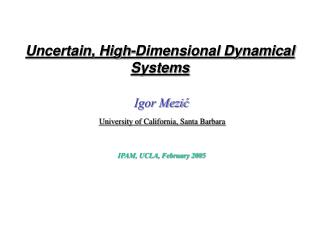DownloadDownload PresentationUncertain, High-Dimensional Dynamical Systems

# Uncertain, High-Dimensional Dynamical Systems

Télécharger la présentation## Uncertain, High-Dimensional Dynamical Systems

- - - - - - - - - - - - - - - - - - - - - - - - - - - E N D - - - - - - - - - - - - - - - - - - - - - - - - - - -
##### Presentation Transcript

1. Uncertain, High-Dimensional Dynamical Systems Igor Mezić University of California, Santa Barbara IPAM, UCLA, February 2005

2. Introduction • Measure of uncertainty? • Uncertainty and spectral theory of dynamical systems. • Model validation and data assimilation. • Decompositions.

3. Dynamical evolution of uncertainty: an example x Output measure Input measure Tradeoff:Bifurcation vs. contracting dynamics

4. Dynamical evolution of uncertainty: general set-up Skew-product system.

5. Dynamical evolution of uncertainty: general set-up

6. Dynamical evolution of uncertainty: general set-up f f T F(z) 1 0

7. Dynamical evolution of uncertainty: simple examples Fw(z) 1 0 Expanding maps: x’=2x

8. Computation of uncertainty in CDF metric

9. Uncertainty in CDF metric: Pitchfork bifurcation x Output measure Input measure

10. Conclusions

11. Introduction PV=NkT • Example: thermodynamics from statistical mechanics “…Any rarified gas will behave that way, no matter how queer the dynamics of its particles…” Goodstein (1985) • Example:Galerkin truncation of evolution equations. • Information comes from a single observable time-series.

12. Introduction When do two dynamical systems exhibit similar behavior?

13. Introduction • Constructive proof that ergodic partitions and invariant • measures of systems can be compared using a • single observable (“Statistical Takens-Aeyels” theorem). • A formalism based on harmonic analysis that extends • the concept of comparing the invariant measure.

14. Set-up Time averages and invariant measures:

15. Set-up

16. Pseudometrics for Dynamical Systems • Pseudometricthat captures statistics: where

17. Ergodic partition

18. Ergodic partition

19. An example: analysis of experimental data

20. Analysis of experimental data

21. Analysis of experimental data

22. Koopman operator, triple decomposition, MOD -Efficient representation of the flow field; can be done with vectors -Lagrangian analysis: FLUCTUATIONS MEAN FLOW PERIODIC APERIODIC Desirable: “Triple decomposition”:

23. Embedding

24. Conclusions • Constructive proof that ergodic partitions and invariant • measures of systems can be compared using a • single observable –deterministic+stochastic. • A formalism based on harmonic analysis that extends • the concept of comparing the invariant measure • Pseudometrics on spaces of dynamical systems. • Statistics – based, linear (but infinite-dimensional).

25. Introduction Everson et al., JPO 27 (1997)

26. Introduction • 4 modes -99% • of the variance! • -no dynamics! Everson et al., JPO 27 (1997)

27. Introduction In this talk: -Account explicitly for dynamics to produce a decomposition. -Tool: lift to infinite-dimensional space of functions on attractor + consider properties of Koopman operator. -Allows for detailed comparison of dynamical properties of the evolution and retained modes. -Split into “deterministic” and “stochastic” parts: useful for prediction purposes.

28. Example: Factors and harmonic analysis

29. Factors and harmonic analysis

30. Harmonic analysis: an example

31. Evolution equations and Koopman operator

32. Evolution equations and Koopman operator Similar:“Wold decomposition”

33. Evolution equations and Koopman operator But how to get this from data?

34. Evolution equations and Koopman operator is almost-periodic. -Remainder has continuous spectrum!

35. Conclusions -Use properties of the Koopman operator to produce a decomposition -Tool: lift to infinite-dimensional space of functions on attractor. -Allows for detailed comparison of dynamical properties of the evolution and retained modes. -Split into “deterministic” and “stochastic” parts: useful for prediction purposes. -Useful for Lagrangian studies in fluid mechanics.

36. -1 0 a1 a2 • But poor for dynamics: Irrationala’s produce the same statistics Invariant measures and time-averages Example:Probability histograms!

37. Dynamical evolution of uncertainty: simple examples • Types of uncertainty: • Epistemic (reducible) • Aleatory (irreducible) • A-priori (initial conditions, • parameters, model structure) • A-posteriori (chaotic dynamics, • observation error) Expanding maps: x’=2x

38. Uncertainty in CDF metric: Examples Uncertainty strongly dependent on distribution of initial conditions.